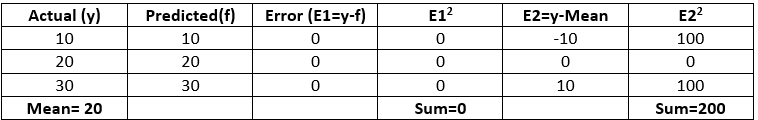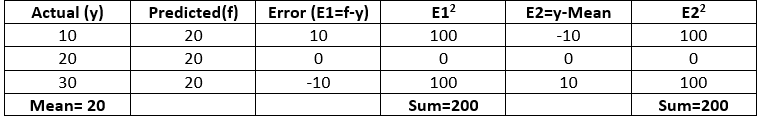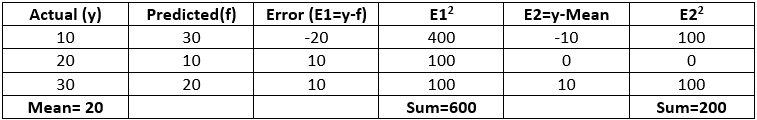# Python – Coefficient of Determination-R2 score

## Python – Coefficient of Determination-R2 score

Coefficient of determination also called as R2 score is used to evaluate the performance of a linear regression model. It is the amount of the variation in the output dependent attribute which is predictable from the input independent variable(s). It is used to check how well-observed results are reproduced by the model, depending on the ratio of total deviation of results described by the model.

Mathematical Formula:

R2= 1- SSres / SStotWhere,
SSres is the sum of squares of the residual errors.
SStot is the total sum of the errors.

Interpretation of R2 score:
Assume R2 = 0.68
It can be referred that 68% of the changeability of the dependent output attribute can be explained by the model while the remaining 32 % of the variability is still unaccounted for.
Rindicates the proportion of data points which lie within the line created by the regression equation. A higher value of R2 is desirable as it indicates better results.

Examples
Case 1 Model gives accurate resultsR2 = 1- 0/200 = 1

Case 2 Model gives same results alwaysR2 = 1- 200/200 = 0

Case 3 Model gives ambiguous resultsR2 = 1- 600/200 = -2

We can import r2_score from sklearn.metrics in Python to compute R2 score.

Python Implementation:
Code 1: Import r2_score from sklearn.metrics

 `from` `sklearn.metrics ``import` `r2_score`

Code 2: Calculate R2 score for all the above cases.

 `### Assume y is the actual value and f is the predicted values` `y ``=``[``10``, ``20``, ``30``]` `f ``=``[``10``, ``20``, ``30``]` `r2 ``=` `r2_score(y, f)` `print``(``'r2 score for perfect model is'``, r2)`

Output:

`r2 score for perfect model is 1.0`
 `### Assume y is the actual value and f is the predicted values` `y ``=``[``10``, ``20``, ``30``]` `f ``=``[``20``, ``20``, ``20``]` `r2 ``=` `r2_score(y, f)` `print``(``'r2 score for a model which predicts mean value always is'``, r2)` ` `

Output:

`r2 score for a model which predicts mean value always is 0.0`

Code 3:

 `### Assume y is the actual value and f is the predicted values` `y ``=` `[``10``, ``20``, ``30``]` `f ``=` `[``30``, ``10``, ``20``]` `r2 ``=` `r2_score(y, f)` `print``(``'r2 score for a worse model is'``, r2)`

Output:

`r2 score for a worse model is -2.0`

Conclusion:

• The best possible score is 1 which is obtained when the predicted values are the same as the actual values.
• R2 score of baseline model is 0.
• During the worse cases, R2 score can even be negative.

Last Updated on March 17, 2022 by admin

## Python – pass multiple arguments to map functionPython – pass multiple arguments to map function

Python – pass multiple arguments to map function The map() function is a built-in function

## Pooling Layer for Convolutional Neural Networks (CNN)Pooling Layer for Convolutional Neural Networks (CNN)

CNN | Introduction to Pooling Layer The pooling operation involves sliding a two-dimensional filter over

## callable() in Pythoncallable() in Python

callable() in Python In general, a callable is something that can be called. This built-in

## Create XML Documents using PythonCreate XML Documents using Python

Create XML Documents using Python Extensible Markup Language(XML), is a markup language that you can

## SHA in PythonSHA in Python

SHA in Python   SHA, ( Secure Hash Algorithms ) are set of cryptographic hash

## Python program to read character by character from a filePython program to read character by character from a file

Python program to read character by character from a file Given a text file. The

## Python – Check if all the values in a list that are greater than a given valuePython – Check if all the values in a list that are greater than a given value

Python | Check if all the values in a list that are greater than a

## Generating all possible Subsequences using RecursionGenerating all possible Subsequences using Recursion

Generating all possible Subsequences using Recursion Given an array. The task is to generate and